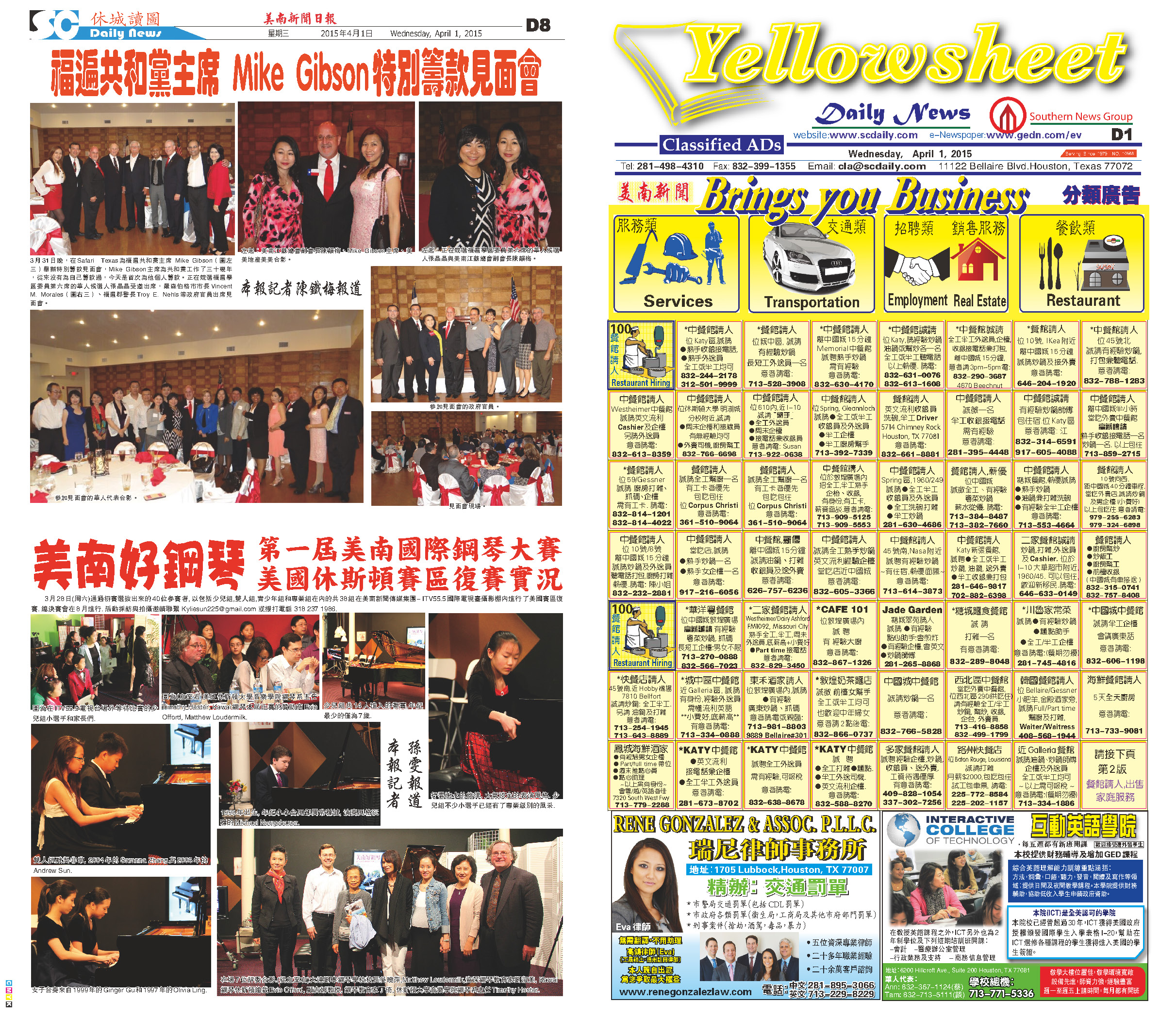150411 Epaper

 A Section B SectionC Section D Section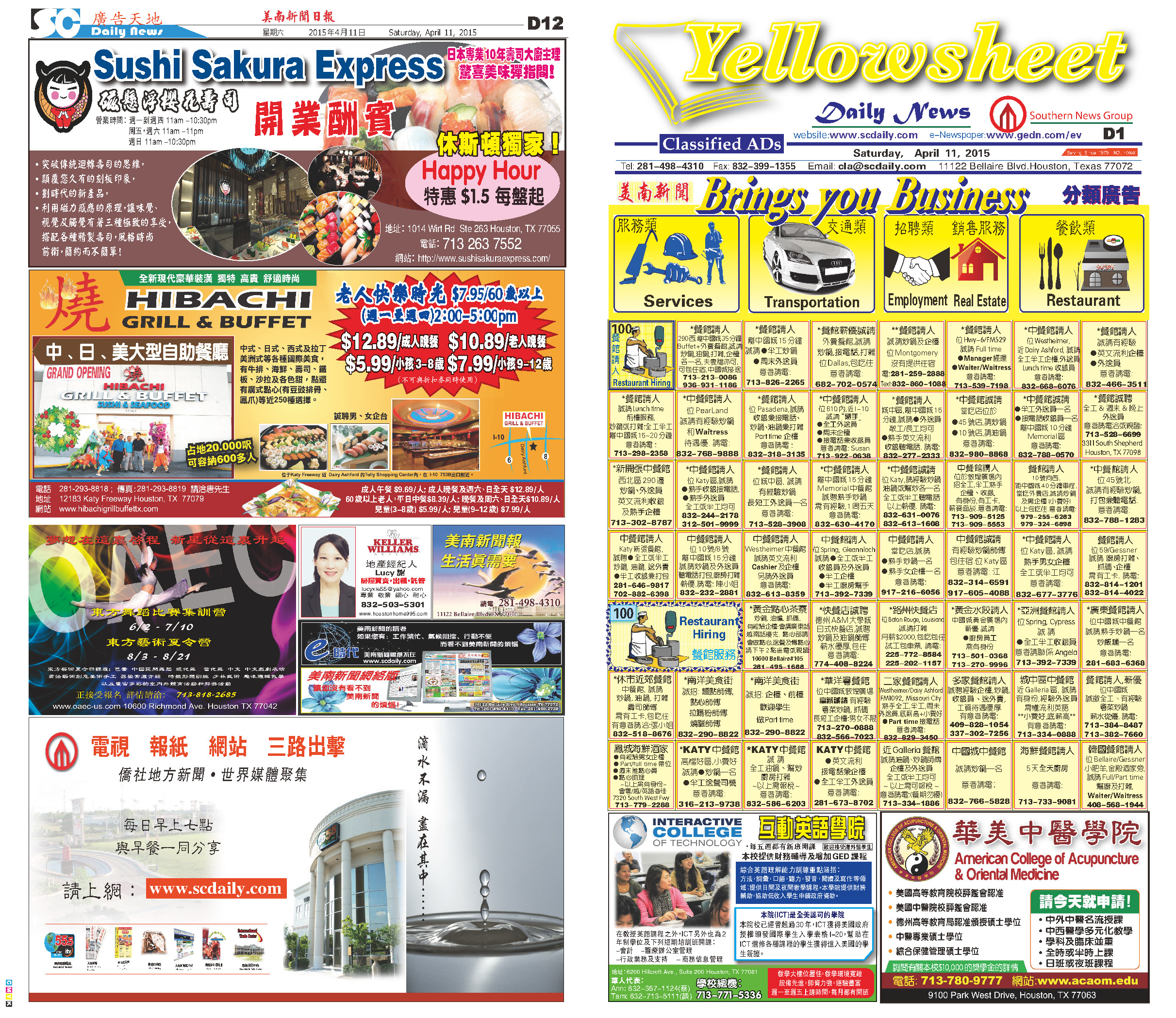150410 Epaper

 A Section B SectionC Section D Section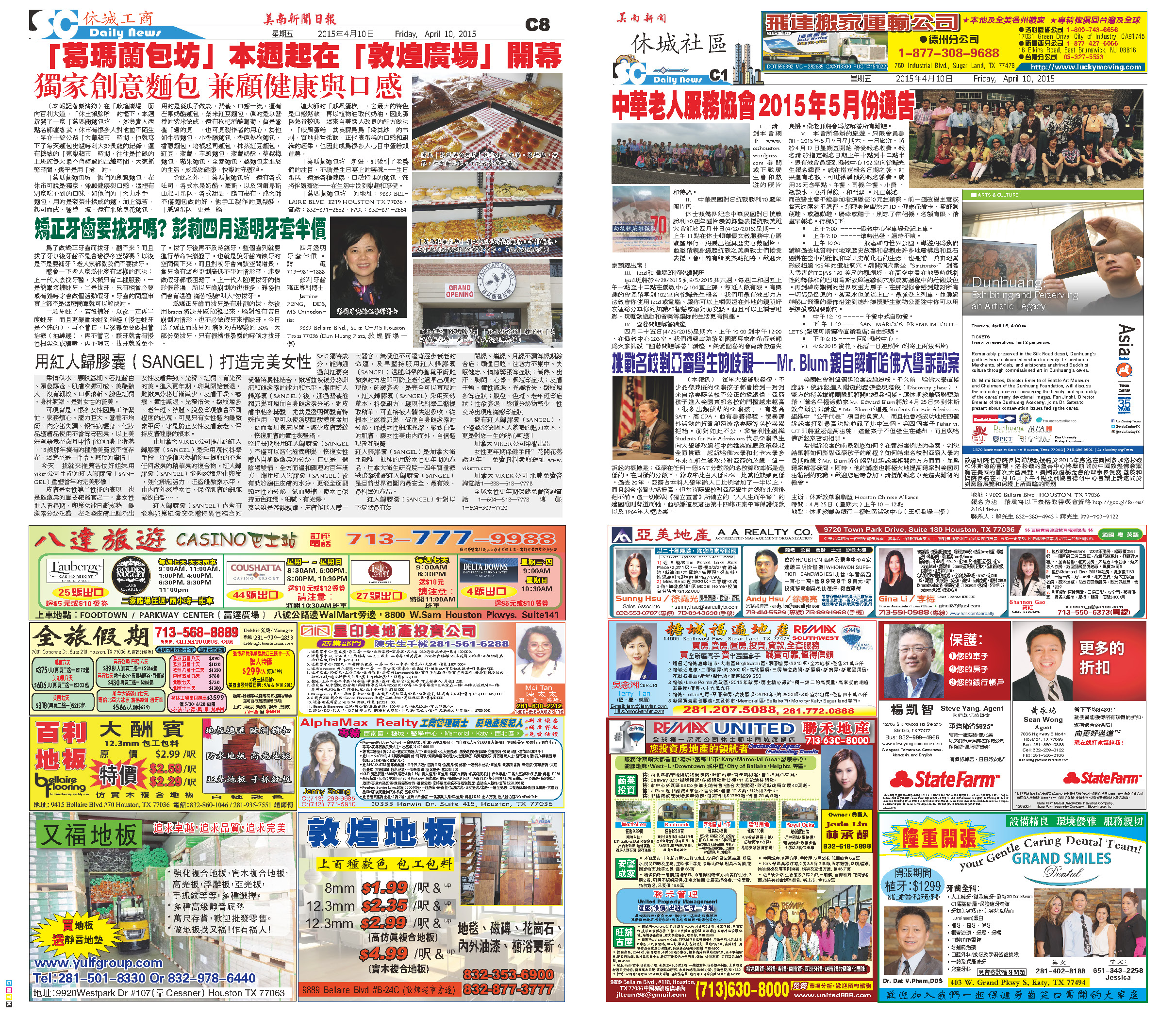150409 Epaper

 A Section B SectionC Section D Section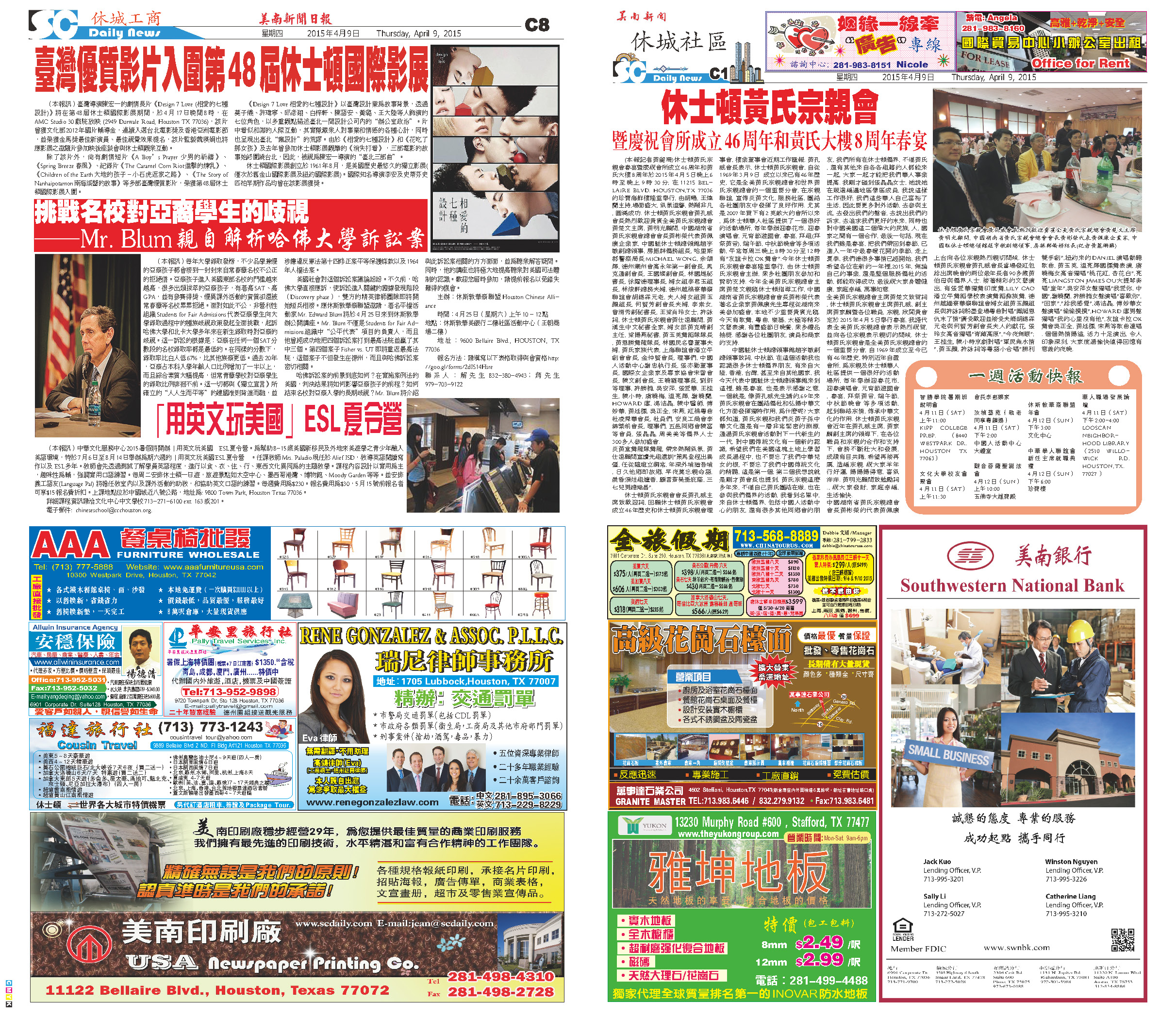150408 Epaper

 A Section B SectionC Section D Section150407 Epaper

 A Section B SectionC Section D Section150406 Epaper

 A Section B SectionC Section D Section150405 Epaper

 A Section B SectionC Section D Section150404 Epaper

 A Section B Section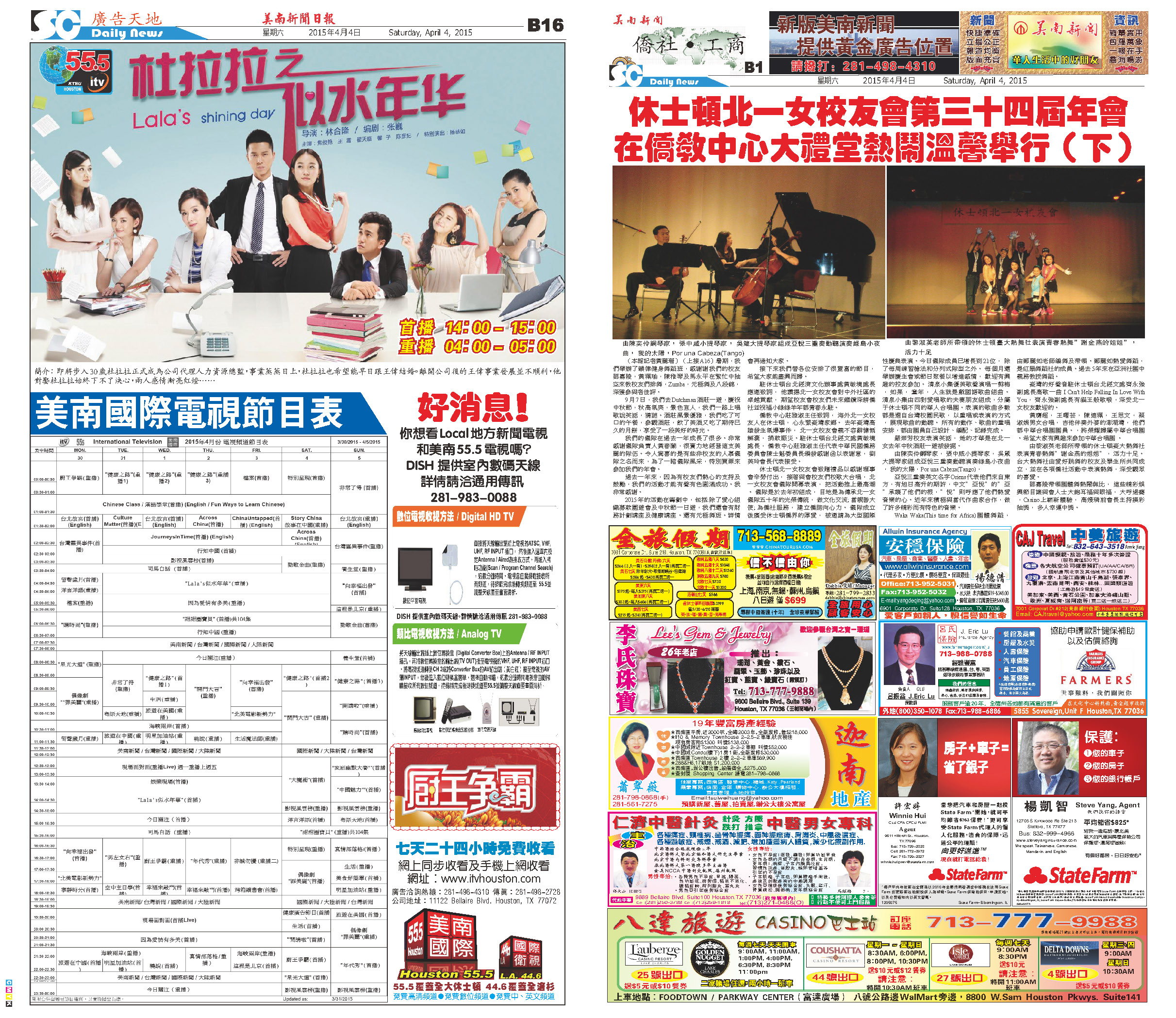C Section D Section150402 Epaper

 A Section B SectionC Section D Section150403 Epaper

 A Section B SectionC Section D Section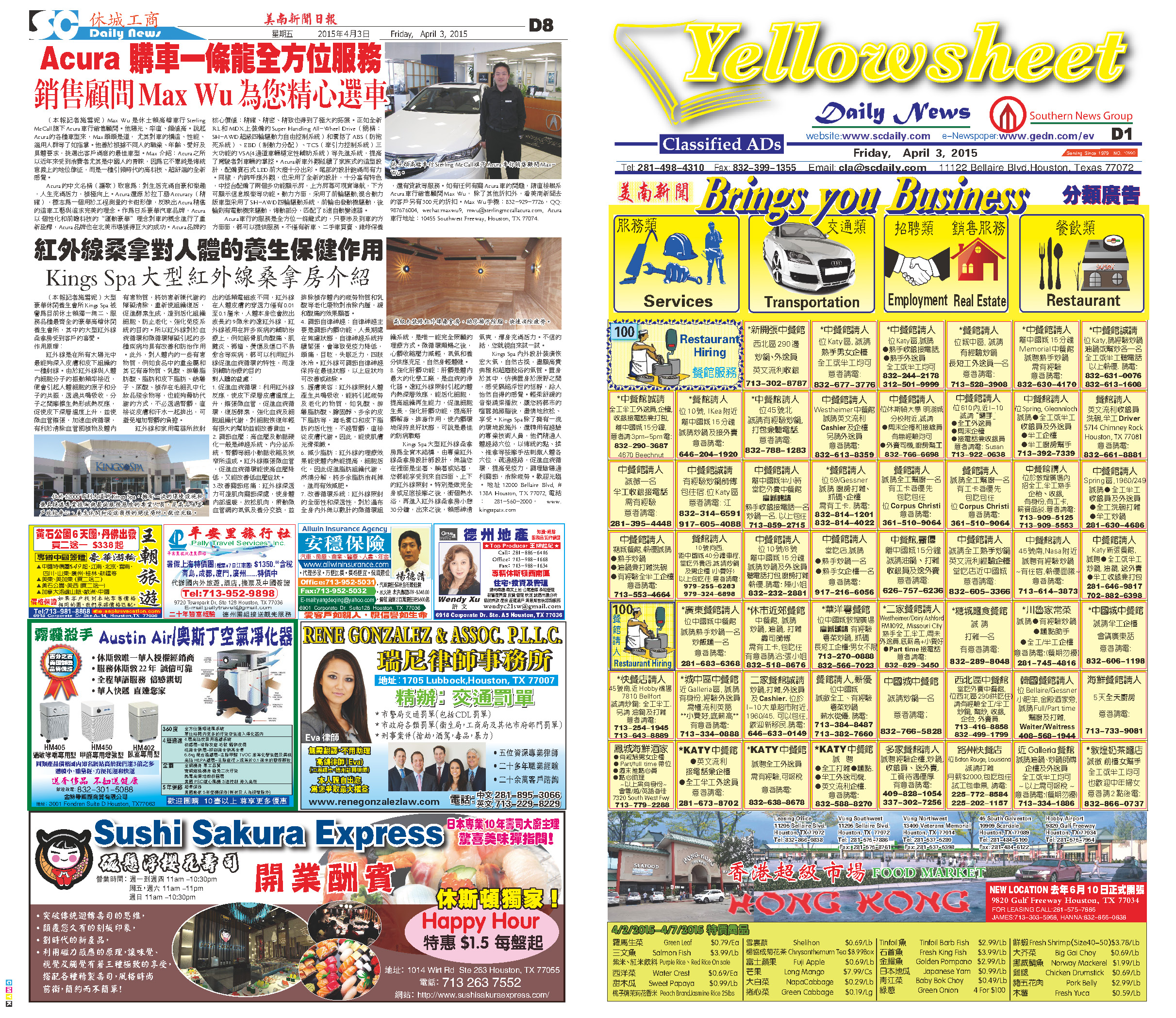150401 Epaper

 A Section B SectionC Section D Section